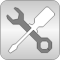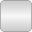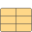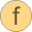# IF97_Utilities

Low level and utility computation for high accuracy water properties according to the IAPWS/IF97 standard# Package ContentsBaseIF97 Modelica Physical Property Model: the new industrial formulation IAPWS-IF97iterwaterBaseProp_ph Intermediate property record for waterwaterBaseProp_ps Intermediate property record for waterrho_props_ps Density as function of pressure and specific entropyrho_ps Density as function of pressure and specific entropyT_props_ps Temperature as function of pressure and specific entropyT_ps Temperature as function of pressure and specific entropyh_props_ps Specific enthalpy as function or pressure and temperatureh_ps Specific enthalpy as function or pressure and temperaturephase_ps Phase as a function of pressure and specific entropyphase_ph Phase as a function of pressure and specific enthalpyphase_dT Phase as a function of pressure and temperaturerho_props_ph Density as function of pressure and specific enthalpyrho_ph Density as function of pressure and specific enthalpyrho_ph_der Derivative function of rho_phT_props_ph Temperature as function of pressure and specific enthalpyT_ph Temperature as function of pressure and specific enthalpyT_ph_der Derivative function of T_phs_props_ph Specific entropy as function of pressure and specific enthalpys_ph Specific entropy as function of pressure and specific enthalpys_ph_der Specific entropy as function of pressure and specific enthalpycv_props_ph Specific heat capacity at constant volume as function of pressure and specific enthalpycv_ph Specific heat capacity at constant volume as function of pressure and specific enthalpyregionAssertReal Assert function for inliningcp_props_ph Specific heat capacity at constant pressure as function of pressure and specific enthalpycp_ph Specific heat capacity at constant pressure as function of pressure and specific enthalpybeta_props_ph Isobaric expansion coefficient as function of pressure and specific enthalpybeta_ph Isobaric expansion coefficient as function of pressure and specific enthalpykappa_props_ph Isothermal compressibility factor as function of pressure and specific enthalpykappa_ph Isothermal compressibility factor as function of pressure and specific enthalpyvelocityOfSound_props_ph Speed of sound as function of pressure and specific enthalpyvelocityOfSound_phisentropicExponent_props_ph Isentropic exponent as function of pressure and specific enthalpyisentropicExponent_ph Isentropic exponent as function of pressure and specific enthalpyddph_props Density derivative by pressureddph Density derivative by pressureddhp_props Density derivative by specific enthalpyddhp Density derivative by specific enthalpywaterBaseProp_pT Intermediate property record for water (p and T preferred states)rho_props_pT Density as function or pressure and temperaturerho_pT Density as function or pressure and temperatureh_props_pT Specific enthalpy as function or pressure and temperatureh_pT Specific enthalpy as function or pressure and temperatureh_pT_der Derivative function of h_pTrho_pT_der Derivative function of rho_pTs_props_pT Specific entropy as function of pressure and temperatures_pT Temperature as function of pressure and temperaturecv_props_pT Specific heat capacity at constant volume as function of pressure and temperaturecv_pT Specific heat capacity at constant volume as function of pressure and temperaturecp_props_pT Specific heat capacity at constant pressure as function of pressure and temperaturecp_pT Specific heat capacity at constant pressure as function of pressure and temperaturebeta_props_pT Isobaric expansion coefficient as function of pressure and temperaturebeta_pT Isobaric expansion coefficient as function of pressure and temperaturekappa_props_pT Isothermal compressibility factor as function of pressure and temperaturekappa_pT Isothermal compressibility factor as function of pressure and temperaturevelocityOfSound_props_pT Speed of sound as function of pressure and temperaturevelocityOfSound_pT Speed of sound as function of pressure and temperatureisentropicExponent_props_pT Isentropic exponent as function of pressure and temperatureisentropicExponent_pT Isentropic exponent as function of pressure and temperaturewaterBaseProp_dT Intermediate property record for water (d and T preferred states)h_props_dT Specific enthalpy as function of density and temperatureh_dT Specific enthalpy as function of density and temperatureh_dT_der Derivative function of h_dTp_props_dT Pressure as function of density and temperaturep_dT Pressure as function of density and temperaturep_dT_der Derivative function of p_dTs_props_dT Specific entropy as function of density and temperatures_dT Temperature as function of density and temperaturecv_props_dT Specific heat capacity at constant volume as function of density and temperaturecv_dT Specific heat capacity at constant volume as function of density and temperaturecp_props_dT Specific heat capacity at constant pressure as function of density and temperaturecp_dT Specific heat capacity at constant pressure as function of density and temperaturebeta_props_dT Isobaric expansion coefficient as function of density and temperaturebeta_dT Isobaric expansion coefficient as function of density and temperaturekappa_props_dT Isothermal compressibility factor as function of density and temperaturekappa_dT Isothermal compressibility factor as function of density and temperaturevelocityOfSound_props_dT Speed of sound as function of density and temperaturevelocityOfSound_dT Speed of sound as function of density and temperatureisentropicExponent_props_dT Isentropic exponent as function of density and temperatureisentropicExponent_dT Isentropic exponent as function of density and temperaturehl_p Compute the saturated liquid specific h(p)hv_p Compute the saturated vapour specific h(p)sl_p Compute the saturated liquid specific s(p)sv_p Compute the saturated vapour specific s(p)rhol_T Compute the saturated liquid d(T)rhov_T Compute the saturated vapour d(T)rhol_p Compute the saturated liquid d(p)rhov_p Compute the saturated vapour d(p)dynamicViscosity Compute eta(d,T) in the one-phase regionthermalConductivity Compute lambda(d,T,p) in the one-phase regionsurfaceTension Compute sigma(T) at saturation TisentropicEnthalpy Isentropic specific enthalpy from p,s (preferably use dynamicIsentropicEnthalpy in dynamic simulation!)isentropicEnthalpy_propsisentropicEnthalpy_der Derivative of isentropic specific enthalpy from p,sdynamicIsentropicEnthalpy Isentropic specific enthalpy from p,s and good guesses of d and T

# Information

This information is part of the Modelica Standard Library maintained by the Modelica Association.

#### Package description:

This package provides high accuracy physical properties for water according to the IAPWS/IF97 standard. It has been part of the ThermoFluid Modelica library and been extended, reorganized and documented to become part of the Modelica Standard library.

An important feature that distinguishes this implementation of the IF97 steam property standard is that this implementation has been explicitly designed to work well in dynamic simulations. Computational performance has been of high importance. This means that there often exist several ways to get the same result from different functions if one of the functions is called often but can be optimized for that purpose.

The original documentation of the IAPWS/IF97 steam properties can freely be distributed with computer implementations, so for curious minds the complete standard documentation is provided with the Modelica properties library. The following documents are included (in directory Modelica/Resources/Documentation/Media/Water/IF97documentation):

• IF97.pdf The standards document for the main part of the IF97.
• Back3.pdf The backwards equations for region 3.
• crits.pdf The critical point data.
• meltsub.pdf The melting- and sublimation line formulation (in IF97_Utilities.BaseIF97.IceBoundaries)
• surf.pdf The surface tension standard definition
• thcond.pdf The thermal conductivity standard definition
• visc.pdf The viscosity standard definition

#### Package contents

• Package BaseIF97 contains the implementation of the IAPWS-IF97 as described in IF97.pdf. The explicit backwards equations for region 3 from Back3.pdf are implemented as initial values for an inverse iteration of the exact function in IF97 for the input pairs (p,h) and (p,s). The low-level functions in BaseIF97 are not needed for standard simulation usage, but can be useful for experts and some special purposes.
• Function water_ph returns all properties needed for a dynamic control volume model and properties of general interest using pressure p and specific entropy enthalpy h as dynamic states in the record ThermoProperties_ph.
• Function water_ps returns all properties needed for a dynamic control volume model and properties of general interest using pressure p and specific entropy s as dynamic states in the record ThermoProperties_ps.
• Function water_dT returns all properties needed for a dynamic control volume model and properties of general interest using density d and temperature T as dynamic states in the record ThermoProperties_dT.
• Function water_pT returns all properties needed for a dynamic control volume model and properties of general interest using pressure p and temperature T as dynamic states in the record ThermoProperties_pT. Due to the coupling of pressure and temperature in the two-phase region, this model can obviously only be used for one-phase models or models treating both phases independently.
• Function hl_p computes the liquid specific enthalpy as a function of pressure. For overcritical pressures, the critical specific enthalpy is returned
• Function hv_p computes the vapour specific enthalpy as a function of pressure. For overcritical pressures, the critical specific enthalpy is returned
• Function sl_p computes the liquid specific entropy as a function of pressure. For overcritical pressures, the critical specific entropy is returned
• Function sv_p computes the vapour specific entropy as a function of pressure. For overcritical pressures, the critical specific entropy is returned
• Function rhol_T computes the liquid density as a function of temperature. For overcritical temperatures, the critical density is returned
• Function rhol_T computes the vapour density as a function of temperature. For overcritical temperatures, the critical density is returned
• Function dynamicViscosity computes the dynamic viscosity as a function of density and temperature.
• Function thermalConductivity computes the thermal conductivity as a function of density, temperature and pressure. Important note: Obviously only two of the three inputs are really needed, but using three inputs speeds up the computation and the three variables are known in most models anyways. The inputs d,T and p have to be consistent.
• Function surfaceTension computes the surface tension between vapour and liquid water as a function of temperature.
• Function isentropicEnthalpy computes the specific enthalpy h(p,s,phase) in all regions. The phase input is needed due to discontinuous derivatives at the phase boundary.
• Function dynamicIsentropicEnthalpy computes the specific enthalpy h(p,s,,dguess,Tguess,phase) in all regions. The phase input is needed due to discontinuous derivatives at the phase boundary. Tguess and dguess are initial guess values for the density and temperature consistent with p and s. This function should be preferred in dynamic simulations where good guesses are often available.

#### Version Info and Revision history

• First implemented: July, 2000 by Hubertus Tummescheit for the ThermoFluid Library with help from Jonas Eborn and Falko Jens Wagner
• Code reorganization, enhanced documentation, additional functions: December, 2002 by Hubertus Tummescheit and moved to Modelica properties library.
Author: Hubertus Tummescheit,
Modelon AB
Ideon Science Park
SE-22370 Lund, Sweden
email: hubertus@modelon.se